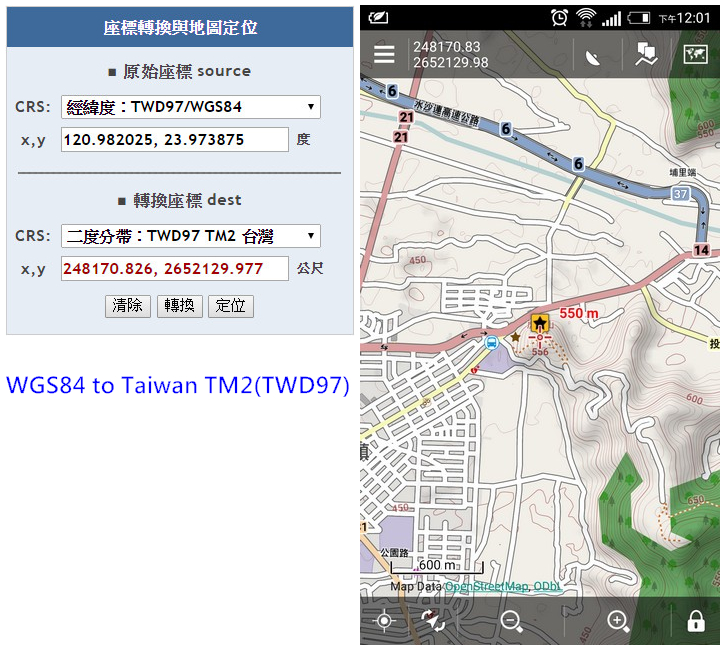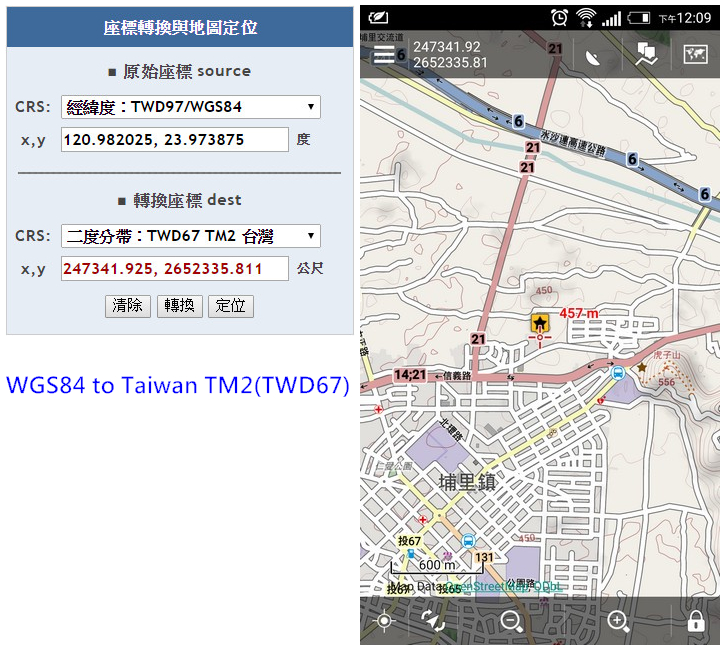# Custom Coordinate Systems for Taiwan TM2

Jing shared this problem 3 years ago
Solved

hi

i read this subject for custom coordinate systems

there is EPSG code for Taiwan TM2 and i add it to config_projections.cfg

1|3825|Penghu TM2(TWD97)|TWD97 TM2 zone 119|Taiwan

1|3826|Taiwan TM2(TWD97)|TWD97 TM2 zone 121|Taiwan

1|3827|Penghu TM2(TWD67)|TWD67 TM2 zone 119|Taiwan

1|3828|Taiwan TM2(TWD67)|TWD67 TM2 zone 121|Taiwan

i check the coords and location by this website (sorry it's only chinese)

without problem when i set in Taiwan TM2(TWD97) or Penghu TM2(TWD97)but wrong location in Taiwan TM2(TWD67) and Penghu TM2(TWD67)1

Good day Jing,

am I wrong or on your screenshots it looks correctly (on both of them) ?1

'think the point should be on same place - on both screenshots

isn't it?1

Ah it isn't, thanks! I was looking just on coordinate values :).1

That's what I mean, they should be in the same position on map.

thanks :)1

Hmm I'm worried I cannot help here.

This is internal configuration that Locus use for transformation

1. # TWD97 / TM2 zone 119
2. <3825> +proj=tmerc +lat_0=0 +lon_0=119 +k=0.9999 +x_0=250000 +y_0=0 +ellps=GRS80 +towgs84=0,0,0,0,0,0,0 +units=m +no_defs <>
3. # TWD97 / TM2 zone 121
4. <3826> +proj=tmerc +lat_0=0 +lon_0=121 +k=0.9999 +x_0=250000 +y_0=0 +ellps=GRS80 +towgs84=0,0,0,0,0,0,0 +units=m +no_defs <>
5. # TWD67 / TM2 zone 119
6. <3827> +proj=tmerc +lat_0=0 +lon_0=119 +k=0.9999 +x_0=250000 +y_0=0 +ellps=aust_SA +units=m +no_defs <>
7. # TWD67 / TM2 zone 121
8. <3828> +proj=tmerc +lat_0=0 +lon_0=121 +k=0.9999 +x_0=250000 +y_0=0 +ellps=aust_SA +units=m +no_defs <>

These parameters are used for Proj.4 library that is used in Locus. So if your web site is correct, and these Proj.4 parameters are also correct (for unknown reason they are not visible on web http://spatialreference.org/ref/epsg/3828/ - tap on proj.4) , then issue should be in Proj.4 library in Locus only.

Because Locus use older version of this lib, solution should be update to new version and if this helps, report to it's developers. Unfortunately update is not currently possible.1

can you fix it If i provide the correct parameters?

this is the parameter I set in QGIS, and i confirm for convert from WGS84 to TWD67 TM2 of coordinate is same that website.

# TWD67 / TM2 zone 119

+proj=tmerc +ellps=GRS67 +towgs84=-752,-358,-179,-.0000011698,.0000018398,.0000009822,.00002329 +lon_0=119 +x_0=250000 +k=0.9999 +to +proj=tmerc +datum=WGS84 +lon_0=119 +x_0=250000 +k=0.9999

# TWD67 / TM2 zone 121

+proj=tmerc +ellps=GRS67 +towgs84=-752,-358,-179,-.0000011698,.0000018398,.0000009822,.00002329 +lon_0=121 +x_0=250000 +k=0.9999 +to +proj=tmerc +datum=WGS84 +lon_0=121 +x_0=250000 +k=0.99991

Configuration of projection changed and seems to work as expected. So you will see in next version.

Thank you for your help!1

This problem that are fixed in 3.9.1

thank you Menion

:)1

Perfect, glad to hear it. Thank you too!